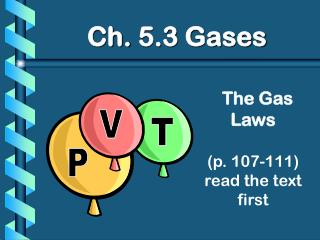# The Gas Laws (p. 107-111) read the text first - PowerPoint PPT PresentationDownload PresentationThe Gas Laws (p. 107-111) read the text first

The Gas Laws (p. 107-111) read the text firstDownload Presentation## The Gas Laws (p. 107-111) read the text first

- - - - - - - - - - - - - - - - - - - - - - - - - - - E N D - - - - - - - - - - - - - - - - - - - - - - - - - - -
##### Presentation Transcript

1. V T P Ch. 5.3 Gases The Gas Laws(p. 107-111)read the text first

2. A. Boyle’s Law P V PV = k

3. A. Boyle’s Law P V • The pressure and volume of a gas are inversely related • at constant mass & temp PV = k

4. A. Boyle’s Law

5. B. Charles’ Law V T • The volume and absolute temperature (K) of a gas are directly related • at constant mass & pressure

6. B. Charles’ Law

7. C. Gay-Lussac’s Law P T • The pressure and absolute temperature (K) of a gas are directly related • at constant mass & volume

8. D. Combined Gas Law P1V1 T1 P2V2 T2 = P T V T PV T PV = k P1V1T2 =P2V2T1

9. E. Gas Law Problems • A gas occupies 473 cm3 at 36°C. Find its volume at 94°C. CHARLES’ LAW GIVEN: V1 = 473 cm3 T1 = 36°C = 309K V2 = ? T2 = 94°C = 367K T V WORK: P1V1T2 = P2V2T1 (473 cm3)(367 K)=V2(309 K) V2 = 562 cm3

10. E. Gas Law Problems • A gas occupies 100. mL at 150. kPa. Find its volume at 200. kPa. BOYLE’S LAW GIVEN: V1 = 100. mL P1 = 150. kPa V2 = ? P2 = 200. kPa P V WORK: P1V1T2 = P2V2T1 (150.kPa)(100.mL)=(200.kPa)V2 V2 = 75.0 mL

11. E. Gas Law Problems • A gas occupies 7.84 cm3 at 71.8 kPa & 25°C. Find its volume at STP. COMBINED GAS LAW P T V GIVEN: V1=7.84 cm3 P1=71.8 kPa T1=25°C = 298 K V2=? P2=101.325 kPa T2=273 K WORK: P1V1T2 = P2V2T1 (71.8 kPa)(7.84 cm3)(273 K) =(101.325 kPa)V2 (298 K) V2 = 5.09 cm3

12. E. Gas Law Problems • A gas’ pressure is 765 torr at 23°C. At what temperature will the pressure be 560. torr? GAY-LUSSAC’S LAW GIVEN: P1 = 765 torr T1 = 23°C = 296K P2 = 560. torr T2 = ? P T WORK: P1V1T2 = P2V2T1 (765 torr)T2 = (560. torr)(309K) T2 = 226 K = -47°C